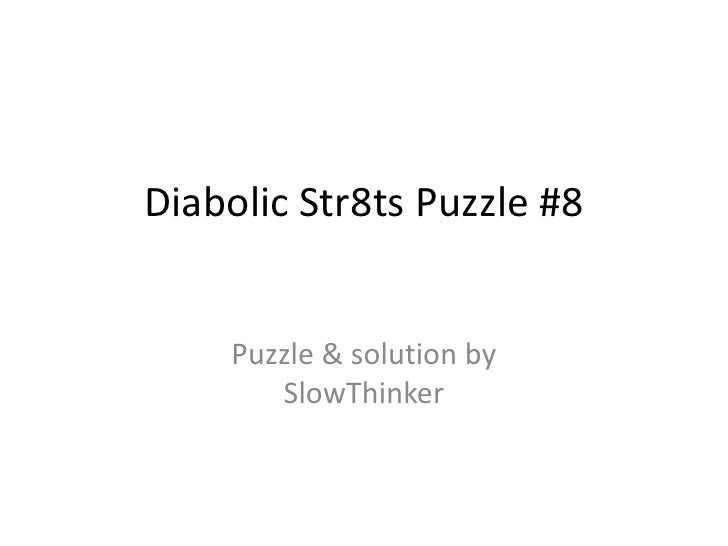Successfully reported this slideshow.
We use your LinkedIn profile and activity data to personalize ads and to show you more relevant ads. You can change your ad preferences anytime.Upcoming SlideShare
×

Diabolic Str8ts #8

1,778 views

Published on

Solution to Diabolic Str8ts #8 puzzle (http://is.gd/slowthinker_diabolic_str8ts_8c) and its tough variation Diabolic #8b (http://is.gd/slowthinker_diabolic_str8ts_8d)

• Full Name
Comment goes here.

Are you sure you want to Yes No
Your message goes here• Be the first to comment

• Be the first to like this

Diabolic Str8ts #8

1. 1. Diabolic Str8ts Puzzle #8 Puzzle & solution by SlowThinker
2. 2. Start positionWith the diabolicStr8ts series, I try topush the boundaries abit. Normal strategiesare not enough tosolve these puzzles.Diabolic #8 is easierthan its variation #8b.So let’s look atDiabolic #8 first. Thestart position is on theright.
3. 3. Unique solutionconstraintIn EF12 we find aunique rectangle on23 which removes 2and 3 from E1.Once E1≠23 FJ1 are ahidden pair on 23.Hence J1=23 andE1=4s.(We will see in Diabolic#8b that E1=4 is crucial forthe easier difficulty of thepuzzle.)
4. 4. Setti on 4Next we can set C6=4due to 4s appearing inall rows.
5. 5. X-Wing on 5Setti on 8HJ35 forms an x-wingon 5 that removes the5s from the yellowfields. This leads toG6=5s.Next with Setti on 8(border marks) we canremove the 5s fromCE8, as 8 has toappear in column 8.
6. 6. X-Wing on 7HJ34 forms an x-wingon 7 that removes the7s from the yellowfields. This leads toG1=7s and D1=6s.This solves quite manyfields.Note that we can alsoremove 1 from EH3,because the x-wing on7 makes 7 a surecandidate in D..J3.
7. 7. X-Wing on 5Another x-wing on 5,this time at CE47 thatresults in E9=6x. Thatin turn solves another5 cells.Next we have a nakedpair BE3=28 thatresults in G3=6p.
8. 8. Chain test:E5=1?At the end we needone chain. Let’s have alook at E5=1:E5=1  H1=1s C1=8, C2=7 Orange: B2=8, B3=2Blue: C7=5, E7=8Which leaves nocandidates in E3.Therefore E5 cannotbe 1.
9. 9. SolutionOnce we know E5=8,the puzzle solveseasily.So this puzzle was nottoo difficult. Let’sexamine its muchtougher variation.
10. 10. Diabolic #8bIn this variation thepuzzle is exactly thesame, but for onechange: instead ofF3=4 we now haveF2=2.As we will see thissmall change greatlyincreases the difficultyof the puzzle.
11. 11. Hidden tripleThe main difference isthat F7 now contains45 as additionalcandidates andtherefore 78 remainsin HJ7. Also, E1 nolonger can be set to 4.EFJ1 now contain thehidden triple 234.
12. 12. Chain test:HJ7=78Let’s test H7=8.H7=8  H1=1 D1=6c  D9=7, D8=8,D7=9.But: with H7=8 D7cannot be 9 as itwould be a strandeddigit. HJ7 ≠ 78.
13. 13. Range test:D..J3=1-6?Let’s assume that D..J3has the range 1-6. J3≠7  J4=7s H4=8With H4=8 we getD9=7 (just like in thetest before)  Thereis no 7 left in row H!Hence D..J3’s rangecannot be 1-6 and wecan remove 1 from theblue fields.
14. 14. Setti on 8Again we can removethe 5s in CE8, as Settion 8 tells us that theremust be a 8 in everycolumn.
15. 15. Test H9=7?H9=7 inhibits an x-wing from forming, solet’s test it:H9=7  H4=8  H1=1H9=7  D9=6  D1=9But this cannot be.The numbers are outof range. Thus H9≠7.Next we get an x-wingon 7 at HJ34.
16. 16. Test C6=1?Next let’s test C6=1using Setti on 4 and 7:C6=1  C12=78S7,E6=4S4  G6=5s G1=7s  C1=8, C2=7,B2=8, B3=2  B4=6C6=1  C8=6c, E6=4S4 C4=35, E1=2 E4=1 (b/c of B4=6)But these twonumbers are out ofrange  C6=4!
17. 17. Test A7=3?As there are only two3s in row A, let’s testA7=3:A7=3  A8=4sA7=3  J7=2  H7=1 HJ8=34So, A7=3 results in acontradiction.Therefore we can setA4=3.
18. 18. Test AB3=12?B3=2  B4=6(a)  J4=7, H4=8 H1=1  C1=78c(b) Because of H1=1 D1=6  D7=9 andbecause B4=6  C4=5we get C7=67Thus we have acontradiction inC12=78 and C78=67.Hence B3=8 and A3=9.Next: X-wing on 8removes 8 from GH5.
19. 19. Test C7=3?There are only two 3sin row C, so let’s testC7=3:C7=3  D7=678 D1=19  C1=7C7=3  J7=2, H7=1 HJ8=43  A8=5 E7=5s  G6=5s G1=7sThis leads to acontradiction  C7≠3which solves a fewmore cells.
20. 20. Test D789=678?D789=678  D1=19D1=19  C1=7 C8=6, C7=5, C4=1 E4=5  E6=79D1=19  G1=6s G3=5  G6=79No 5 left in EFG6 C789 ≠ 678  C7=9Plus: we can removeG6=6 as this againyields D1=19. Thus weknow that D1=6s!
21. 21. Test E5=1?There are only two 1sin column 5  let’stest E5=1. The greencells solve quickly:E5=1  E6=9s, E7=8s,F5=8s, F6=7, F7=6From there:F7=6  B7=4  B5=6 J5=5F7=6  F9=5  H9=4 H5=5Contradiction, thus:H5=1s & E4=1s!
22. 22. SolutionFinally this solves thepuzzle. The mainobstacle was that E1was undeterminedand not equal to 4, asin the easy variation.There are of courseother ways to solvethis puzzle. I tried toshow tests & chainsthat are not too long.
23. 23. GlossaryLetters appended to steps indicate the last strategy used, just before filling in a field:• No letter … number was last candidate in field• s … single (last) candidate for that number in compartment• c … compartment range check• d … stranded (unreachable/impossible) digits removed• h … high/low range check across compartments• p/t/q … naked pair / naked triple / naked quadruple• ph/th/qh … hidden pair / hidden triple / hidden quadruple• x … X-wing (2 rows / 2 columns)• w … Swordfish (3 rows / 3 columns)• j … Jellyfish (4 rows / 4 columns)• L … large gap field• Sx … Setti’s rule (count the numbers rule) – ‘x’ is the analysed number• u … unique rectangle• y … Y-Wing or XY-chains
24. 24. Diabolic Str8ts Puzzle #8Solution by SlowThinkerNote: there are other (maybe easier) ways to solve this puzzle.View & download my strategy slides from:http://slideshare.net/SlowThinker/str8ts-basic-and-advanced-strategiesor from Google Docs:http://is.gd/slowthinker_str8ts_strategy